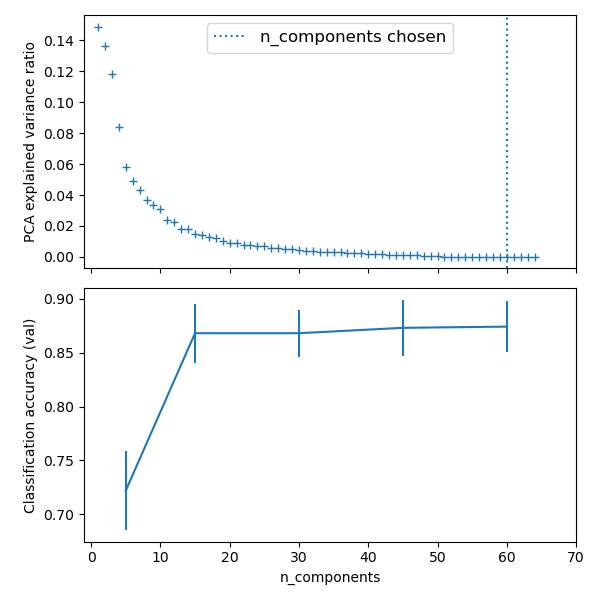# Putting it all together¶

## Pipelining¶

We have seen that some estimators can transform data and that some estimators can predict variables. We can also create combined estimators:

from sklearn.decomposition import PCA
from sklearn.linear_model import LogisticRegression
from sklearn.pipeline import Pipeline
from sklearn.model_selection import GridSearchCV
from sklearn.preprocessing import StandardScaler

# Define a pipeline to search for the best combination of PCA truncation
# and classifier regularization.
pca = PCA()
# Define a Standard Scaler to normalize inputs
scaler = StandardScaler()

# set the tolerance to a large value to make the example faster
logistic = LogisticRegression(max_iter=10000, tol=0.1)
pipe = Pipeline(steps=[("scaler", scaler), ("pca", pca), ("logistic", logistic)])

# Parameters of pipelines can be set using '__' separated parameter names:
param_grid = {
"pca__n_components": [5, 15, 30, 45, 60],
"logistic__C": np.logspace(-4, 4, 4),
}
search = GridSearchCV(pipe, param_grid, n_jobs=2)
search.fit(X_digits, y_digits)
print("Best parameter (CV score=%0.3f):" % search.best_score_)
print(search.best_params_)

# Plot the PCA spectrum
pca.fit(X_digits)

fig, (ax0, ax1) = plt.subplots(nrows=2, sharex=True, figsize=(6, 6))
ax0.plot(
np.arange(1, pca.n_components_ + 1), pca.explained_variance_ratio_, "+", linewidth=2
)
ax0.set_ylabel("PCA explained variance ratio")

ax0.axvline(
search.best_estimator_.named_steps["pca"].n_components,
linestyle=":",
label="n_components chosen",
)## Face recognition with eigenfaces¶

The dataset used in this example is a preprocessed excerpt of the “Labeled Faces in the Wild”, also known as LFW:

"""
===================================================
Faces recognition example using eigenfaces and SVMs
===================================================

The dataset used in this example is a preprocessed excerpt of the
"Labeled Faces in the Wild", aka LFW_:

http://vis-www.cs.umass.edu/lfw/lfw-funneled.tgz (233MB)

.. _LFW: http://vis-www.cs.umass.edu/lfw/

"""
# %%
from time import time
import matplotlib.pyplot as plt

from sklearn.model_selection import train_test_split
from sklearn.model_selection import RandomizedSearchCV
from sklearn.datasets import fetch_lfw_people
from sklearn.metrics import classification_report
from sklearn.metrics import ConfusionMatrixDisplay
from sklearn.preprocessing import StandardScaler
from sklearn.decomposition import PCA
from sklearn.svm import SVC
from sklearn.utils.fixes import loguniform

# %%

lfw_people = fetch_lfw_people(min_faces_per_person=70, resize=0.4)

# introspect the images arrays to find the shapes (for plotting)
n_samples, h, w = lfw_people.images.shape

# for machine learning we use the 2 data directly (as relative pixel
# positions info is ignored by this model)
X = lfw_people.data
n_features = X.shape

# the label to predict is the id of the person
y = lfw_people.target
target_names = lfw_people.target_names
n_classes = target_names.shape

print("Total dataset size:")
print("n_samples: %d" % n_samples)
print("n_features: %d" % n_features)
print("n_classes: %d" % n_classes)

# %%
# Split into a training set and a test and keep 25% of the data for testing.

X_train, X_test, y_train, y_test = train_test_split(
X, y, test_size=0.25, random_state=42
)

scaler = StandardScaler()
X_train = scaler.fit_transform(X_train)
X_test = scaler.transform(X_test)

# %%
# Compute a PCA (eigenfaces) on the face dataset (treated as unlabeled
# dataset): unsupervised feature extraction / dimensionality reduction

n_components = 150

print(
"Extracting the top %d eigenfaces from %d faces" % (n_components, X_train.shape)
)
t0 = time()
pca = PCA(n_components=n_components, svd_solver="randomized", whiten=True).fit(X_train)
print("done in %0.3fs" % (time() - t0))

eigenfaces = pca.components_.reshape((n_components, h, w))

print("Projecting the input data on the eigenfaces orthonormal basis")
t0 = time()
X_train_pca = pca.transform(X_train)
X_test_pca = pca.transform(X_test)
print("done in %0.3fs" % (time() - t0))

# %%
# Train a SVM classification model

print("Fitting the classifier to the training set")
t0 = time()
param_grid = {
"C": loguniform(1e3, 1e5),
"gamma": loguniform(1e-4, 1e-1),
}
clf = RandomizedSearchCV(
SVC(kernel="rbf", class_weight="balanced"), param_grid, n_iter=10
)
clf = clf.fit(X_train_pca, y_train)
print("done in %0.3fs" % (time() - t0))
print("Best estimator found by grid search:")
print(clf.best_estimator_)

# %%
# Quantitative evaluation of the model quality on the test set

print("Predicting people's names on the test set")
t0 = time()
y_pred = clf.predict(X_test_pca)
print("done in %0.3fs" % (time() - t0))

print(classification_report(y_test, y_pred, target_names=target_names))
ConfusionMatrixDisplay.from_estimator(
clf, X_test_pca, y_test, display_labels=target_names, xticks_rotation="vertical"
)
plt.tight_layout()
plt.show()

# %%
# Qualitative evaluation of the predictions using matplotlib

def plot_gallery(images, titles, h, w, n_row=3, n_col=4):
"""Helper function to plot a gallery of portraits"""
plt.figure(figsize=(1.8 * n_col, 2.4 * n_row))
for i in range(n_row * n_col):
plt.subplot(n_row, n_col, i + 1)
plt.imshow(images[i].reshape((h, w)), cmap=plt.cm.gray)
plt.title(titles[i], size=12)
plt.xticks(())
plt.yticks(())

# %%
# plot the result of the prediction on a portion of the test set

def title(y_pred, y_test, target_names, i):
pred_name = target_names[y_pred[i]].rsplit(" ", 1)[-1]
true_name = target_names[y_test[i]].rsplit(" ", 1)[-1]
return "predicted: %s\ntrue:      %s" % (pred_name, true_name)

prediction_titles = [
title(y_pred, y_test, target_names, i) for i in range(y_pred.shape)
]

plot_gallery(X_test, prediction_titles, h, w)
# %%
# plot the gallery of the most significative eigenfaces

eigenface_titles = ["eigenface %d" % i for i in range(eigenfaces.shape)]
plot_gallery(eigenfaces, eigenface_titles, h, w)

plt.show()

# %%
# Face recognition problem would be much more effectively solved by training
# convolutional neural networks but this family of models is outside of the scope of
# the scikit-learn library. Interested readers should instead try to use pytorch or
# tensorflow to implement such models.


Expected results for the top 5 most represented people in the dataset:

                   precision    recall  f1-score   support

Gerhard_Schroeder       0.91      0.75      0.82        28
Donald_Rumsfeld       0.84      0.82      0.83        33
Tony_Blair       0.65      0.82      0.73        34
Colin_Powell       0.78      0.88      0.83        58
George_W_Bush       0.93      0.86      0.90       129

avg / total       0.86      0.84      0.85       282


## Open problem: Stock Market Structure¶

Can we predict the variation in stock prices for Google over a given time frame?

Learning a graph structure